### Home > CALC > Chapter 7 > Lesson 7.4.4 > Problem7-210

7-210.
1. Multiple Choice: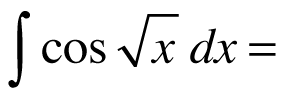Homework Help ✎

1. sin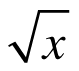+ C

2. 2x sin x − 2 sin x + C

3. 2sin+ 2 cos+ C

4. 2x cos x − 2 sin x + C

5. 2cos+ 2 cos+ C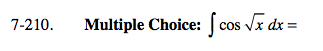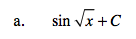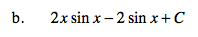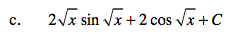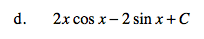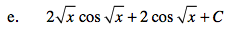First use u-Sub:

Then use integration by parts:

$U=\sqrt{x}$

$\frac{dU}{dx}=\frac{1}{2\sqrt{x}}$

$2\sqrt{x}dU=dx=2UdU$

$\int 2U\text{cos}(U)dU$

Let f(x) = 2U and dg = cos(U)dU
Find df = __________ and g(x) = ____________.

$\text{Then evaluate: }f(x)g(x)-\int g(x)df$